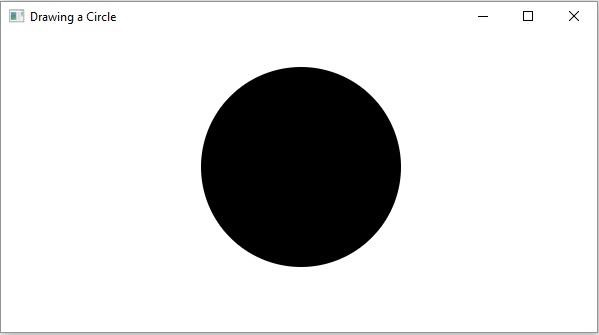# How to draw a geometrical 2D shape in JavaFX?

In general, a 2D shape is a geometrical figure that can be drawn on the XY plane, these include Line, Rectangle, Circle, etc.

The javafx.scene.shape package provides you, various classes, each of them represents/defines a 2d geometrical object or, an operation on them. The class named Shape is the base class of all the 2-Dimensional shapes in JavaFX.

## Creating 2D shapes

To draw a 2D geometrical shape using JavaFX you need to −

• Instantiate the class − Instantiate the respective class. For example, if you want to draw a circle you need to instantiate the Circle class as shown below −

//Drawing a Circle
Circle circle = new Circle();
• Set the properties − Set properties of the shape using the method of its respective class. For example, To draw a circle you need the center and radius and you can set them using the setCenterX(), setCenterY() and setRadius() methods respectively.

//Setting the properties of the circle
circle.setCenterX(300.0f);
circle.setCenterY(135.0f);
circle.setRadius(100.0f);
• Add the shape object to the group − Finally, pass the shape created as a parameter to the group constructor as −

Group root = new Group(circle);

## Example

import javafx.application.Application;
import javafx.scene.Group;
import javafx.scene.Scene;
import javafx.stage.Stage;
import javafx.scene.shape.Circle;
public class CircleExample extends Application {
public void start(Stage stage) {
//Drawing a Circle
Circle circle = new Circle();
//Setting the properties of the circle
circle.setCenterX(300.0f);
circle.setCenterY(135.0f);
//Creating a Group object
Group root = new Group(circle);
//Creating a scene object
Scene scene = new Scene(root, 600, 300);
//Setting title to the Stage
stage.setTitle("Drawing a Circle");
stage.setScene(scene);
//Displaying the contents of the stage
stage.show();
}
public static void main(String args[]){
launch(args);
}
}

## Output